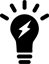### Version:

7.0.1
Copy Doc ID c672b7cf-ea72-11eb-97f7-00505692583a:834824

## CAPWAP bandwidth formula

The following section provides information on how to calculate the control plane CAPWAP traffic load in local bridging. The formula provided can help estimate the approximate package bandwidth cost. This is important for knowing precisely how much bandwidth is required on a WAN link for a centralized FortiGate managing hundreds of access points.

There are multiple factors that might affect the volume of CAPWAP control traffic, including the number of stations there are and large WiFi events.

The tables below depict basic and commonly used optional CAPWAP bandwidth costs, on a per-AP basis.

Note the following:

• STA: The number of stations associated with the FortiAP.
• ARP scan: Finds hidden devices in your network.
• VAP: The number of VAPS held by the FortiAP.
• Radio: The number of radios (maximum of two) enabled by the FortiAP.
###### Basic per-AP CAPWAP bandwidth costs

Content

Time (seconds)

Package bandwidth cost (bps)

Echo Req

30

16

(66+16)*8/30=21.86

STA scan

30

25+20*sta

(66+25+20*sta)*8/30=24.26+5.3*sta

ARP scan

30

25+18*sta

(66+25+18*sta)*8/30=24.26+4.8*sta

STA CAP

30

25+19*sta

(66+25+19*sta)*8/30=24.26+5.1*sta

STA stats

1

25+41*sta

(66+25+41*sta)*8/1=728.0+328.0*sta

VAP stats

15

40+18*vap

(66+40+18*vap)*8/15=56.53+9.6*vap

15

Total:

###### Commonly used optional per-AP CAPWAP bandwidth costs

Content

Time (seconds)

Package bandwidth cost (bps)

AP scan

30

25+63*scanned-ap

(66+25+63*scanned-ap)*8/30=24.26+16.8*scanned-ap

Total:Enabling WIDS features, LLDP, MESH, FortiPresence, and Client Station Locating Service can lead to additional bandwidth consumption.
###### Example:

There are 100 FortiAPs, with 187 stations distributed among them. Each FortiAP holds five VAPs among their radios, and each enables two radios. The basic CAPWAP bandwidth cost would be:

908.7*100+343.2*187+9.6*5*100+13.3*2*100 = 162.51 kbps

Additionally, if two FortiAPs enabled "AP scan", and suppose one scans 99 APs in each scan and the other scans 20 APs in each scan, the additional CAPWAP bandwidth cost would be:

(24.26+16.8*99)+(24.26+16.8*20) = 2 kbps

### LLDP protocol

The LLDP protocol is enabled by default when you create a new FortiAP profile. Each FortiAP using that profile can then send back information about the switch and port that it is connected to. You can also manage the LLDP protocol in the FortiAP Profile via the CLI.

To enable LLDP, enter the following:

config wireless-controller wtp-profile

edit <profile-name>

set lldp enable

end

## CAPWAP bandwidth formula

The following section provides information on how to calculate the control plane CAPWAP traffic load in local bridging. The formula provided can help estimate the approximate package bandwidth cost. This is important for knowing precisely how much bandwidth is required on a WAN link for a centralized FortiGate managing hundreds of access points.

There are multiple factors that might affect the volume of CAPWAP control traffic, including the number of stations there are and large WiFi events.

The tables below depict basic and commonly used optional CAPWAP bandwidth costs, on a per-AP basis.

Note the following:

• STA: The number of stations associated with the FortiAP.
• ARP scan: Finds hidden devices in your network.
• VAP: The number of VAPS held by the FortiAP.
• Radio: The number of radios (maximum of two) enabled by the FortiAP.
###### Basic per-AP CAPWAP bandwidth costs

Content

Time (seconds)

Package bandwidth cost (bps)

Echo Req

30

16

(66+16)*8/30=21.86

STA scan

30

25+20*sta

(66+25+20*sta)*8/30=24.26+5.3*sta

ARP scan

30

25+18*sta

(66+25+18*sta)*8/30=24.26+4.8*sta

STA CAP

30

25+19*sta

(66+25+19*sta)*8/30=24.26+5.1*sta

STA stats

1

25+41*sta

(66+25+41*sta)*8/1=728.0+328.0*sta

VAP stats

15

40+18*vap

(66+40+18*vap)*8/15=56.53+9.6*vap

15

Total:

###### Commonly used optional per-AP CAPWAP bandwidth costs

Content

Time (seconds)

Package bandwidth cost (bps)

AP scan

30

25+63*scanned-ap

(66+25+63*scanned-ap)*8/30=24.26+16.8*scanned-ap

Total:Enabling WIDS features, LLDP, MESH, FortiPresence, and Client Station Locating Service can lead to additional bandwidth consumption.
###### Example:

There are 100 FortiAPs, with 187 stations distributed among them. Each FortiAP holds five VAPs among their radios, and each enables two radios. The basic CAPWAP bandwidth cost would be:

908.7*100+343.2*187+9.6*5*100+13.3*2*100 = 162.51 kbps

Additionally, if two FortiAPs enabled "AP scan", and suppose one scans 99 APs in each scan and the other scans 20 APs in each scan, the additional CAPWAP bandwidth cost would be:

(24.26+16.8*99)+(24.26+16.8*20) = 2 kbps

### LLDP protocol

The LLDP protocol is enabled by default when you create a new FortiAP profile. Each FortiAP using that profile can then send back information about the switch and port that it is connected to. You can also manage the LLDP protocol in the FortiAP Profile via the CLI.

To enable LLDP, enter the following:

config wireless-controller wtp-profile

edit <profile-name>

set lldp enable

end